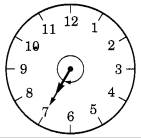# Free Ncert Class 6 Math Understanding Elementary Shapes Exercise 5.2

## Understanding Elementary Shapes Class 6 Ex. 5.2

New NCERT Class 6 Math Free Solution.

Exercise 5.2

Question 1 :- What fraction of a clockwise revolution does the hour hand of a clock turn through, when it goes from
(a) 3 to 9
(b) 4 to 7
(c) 7 to 10
(d) 12 to 9
(e) 1 to 10
(f) 6 to 3
Solution 1:-

(a) 3 to 9
9 – 3 = 6
6/12 = 1/2 of a revolution or two right angles.

(b) 4 to 7
7 – 4 = 3
3/12 = 1/4 of a revolution or one right angle.

(c) 7 to 10
10 – 7 = 3
3/12 = 1/4 of a revolution or one right angle.

(d) 12 to 9 i.e., 12 to 6 and then 6 to 9
12 to 6; 12 – 6 = 6 and 6 to 9; 9 – 6 = 3
6 + 3 = 9;
9/12 = 3/4 of a revolution or three right angle.

(e) 1 to 10
10 – 1 = 9
9/12 = 3/4 of a revolution or three right angle.

(f) 6 to 3  or  6 to 12 and then 12 to 3
6 to 12 = 12 – 6 = 6 and 12 to 3 = 0 to 3 = 3 – 0 = 3
6 + 3 = 9;
9/12 = 3/4 of a revolution or three right angle.

Question 2 :- Where will the hand of a clock stop if it
(a) starts at 12 and makes 1/2 of a revolution, clockwise?
(b) starts at 2 and makes 1/2 of a revolution, clockwise?
(c) starts at 5 and makes 1/4 of a revolution, clockwise?
(d) starts at 5 and makes 3/4 of a revolution, clockwise?
Solution 2:-

(a) Starting from 12 and making 1/2 of a revolution, the clock hand stops at 6.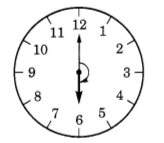(b)The clock hand starts at 2 and completes 1/2 of a revolution before stopping at 8.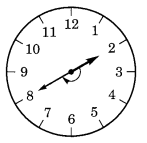(c) Starting from 5 and making 1/4 of a revolution, the clock hand stops at 8.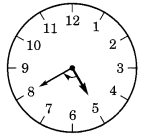(d) Starting from 5 and making 3/4 of a revolution, the clock hand stops at 2.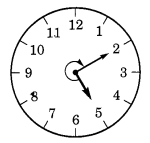Question 3 :- Which direction will you face if you start facing
(a) east and make 1/2 of a revolution clockwise?
(b ) east and make 3/2 of a revolution clockwise?
(c) west and make 3/4 of a revolution anticlockwise?
(d) south and make one full revolution?
(Should we specify clockwise or anti-clockwise for this last question? Why not?)

Solution 3:- (a) West    (b)   West   (c) North   (d) South.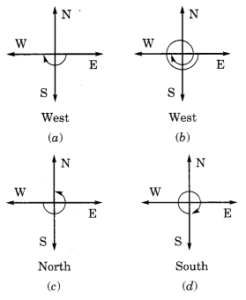Taking one full revolution we will reach back to the original (starting) position.
Therefore, it is irrelevant whether we go in a clockwise or counterclockwise direction.Question 4 :- What part of a revolution have you turned through if you stand facing
(a) east and turn clockwise to face north?
(b) south and turn clockwise to face east?
(c) west and turn clockwise to face east?
Solution 4:-

(a) It takes 3/4 of a revolution to travel from east to north in a clockwise direction.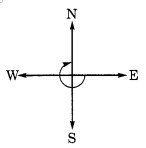(b) It takes 3/4 of a revolution to face east if we turn clockwise from the south pole.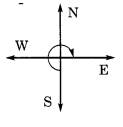(c) It takes half a rotation to face east if we turn clockwise from west to east.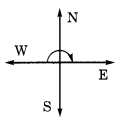Question 5 :- Find the number of right angles turned through by the hour hand of a clock when it goes from
(a) 3 to 6
(b) 2 to 8
(c) 5 to 11
(d) 10 to 1
(e) 12 to 9
(f) 12 to 6
Solution 5:-

(a) 3 to 6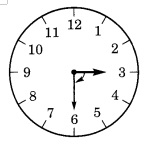The hour hand rotates through 1 right angle from 3 to 6.

(b) 2 to 8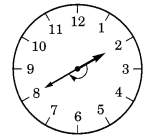The hour hand rotates through 2 right angle from 2 to 8.

(c) 5 to 11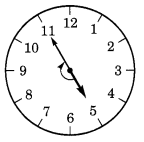The hour hand rotates through 2 right angle from 5 to 11.

(d) 10 to 1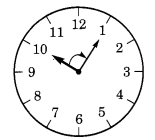The hour hand rotates through 1 right angle from 10 to 1.

(e) 12 to 9The hour hand rotates through 3 right angle from 12 to 9.

(f) 12 to 6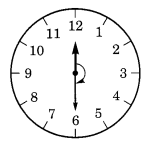The hour hand rotates through 2 right angle from 12 to 6.

Question 6 :- How many right angles do you make if you start facing
(a) south and turn clockwise to west?
(b) north and turn anticlockwise to east?
(c) west and turn to west?
(d) south and turn to north?
Solution 6:-

(a) One     (b) Three      (c) Four     (d) Two (clockwise or anti-clockwise).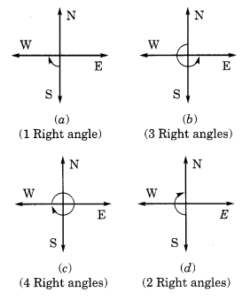Question 7 :- Where will the hour hand of a clock stop if it starts
(a) from 6 and turns through 1 right angle?
(b) from 8 and turns through 2 right angles?
(c) from 10 and turns through 3 right angles?
(d) from 7 and turns through 2 straight angles?
Solution 7:- (we should consider only clockwise direction here.)

(a)The hour hand begins at 6 and stops at 9 after making 1 right angle turn.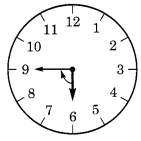(b) The hour hand begins at 8 and stops at 2 after making 2 right angle turn.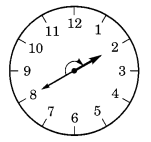(c) The hour hand begins at 10 and stops at 7 after making 3 right angle turn.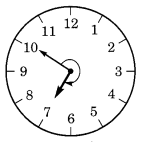(d) The hour hand begins at 7 and stops at 7 after making 2 straight angles turn.